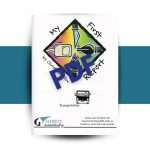Previous\$45.95
Next

#### My First Report: Transportation PDF

\$5.95# Math-It

\$55.95

A fresh and creative approach to the basic skill of computation

• Reduction of voluminous memory facts to simple rules
• Chance to be far more skillful in numbers than most adults
• Ability to easily add 3-digit numbers in one breath
• Simple and ingenious techniques to computing math problems
• Ability to add large columns of numbers quickly
• A fun way to remove the blocks to learning basic math
• Shortcut skills for various math functions as in the following example:Did you know that the 11s times table is easy to figure in your head?Here’s the trick: When you multiply 63 by 11 put a space between the 6 and the 3, add the 6 and 3 together, and place their sum (9) in the space. The answer is 693. Now try multiplying 43 by 11 this way. Did you get 473?

Math-It is a program designed by Elmer Brooks to teach basic facts of addition, subtraction, and multiplication. Full of “tricks” to help students become whizzes in the basics. Children who can count back from 20 as they can tie their shoes with their eyes closed are ready for Math-It. Comes in a case and includes two compact discs, one which guides the student through the game and another with pdf file of How Stevie Learned His Math and Math-It Guidebook; game board; cards for Timzit, Dubblit, and Addit.

Out of stock

SKU: 1717 Categories: , , , Tags: , , , , , ,

## Description

A fresh and creative approach to the basic skill of computation

• Reduction of voluminous memory facts to simple rules
• Chance to be far more skillful in numbers than most adults
• Ability to easily add 3-digit numbers in one breath
• Simple and ingenious techniques to computing math problems
• Ability to add large columns of numbers quickly
• A fun way to remove the blocks to learning basic math
• Shortcut skills for various math functions as in the following example:Did you know that the 11s times table is easy to figure in your head?Here’s the trick: When you multiply 63 by 11 put a space between the 6 and the 3, add the 6 and 3 together, and place their sum (9) in the space. The answer is 693. Now try multiplying 43 by 11 this way. Did you get 473?

Math-It is a program designed by Elmer Brooks to teach basic facts of addition, subtraction, and multiplication. Full of “tricks” to help students become whizzes in the basics. Children who can count back from 20 as they can tie their shoes with their eyes closed are ready for Math-It. Comes in a case and includes two compact discs, one which guides the student through the game and another with pdf file of How Stevie Learned His Math and Math-It Guidebook; game board; cards for Timzit, Dubblit, and Addit.

window.dataLayer = window.dataLayer || [];
function gtag(){dataLayer.push(arguments);}
gtag(‘js’, new Date());

gtag(‘config’, ‘AW-663197381’);